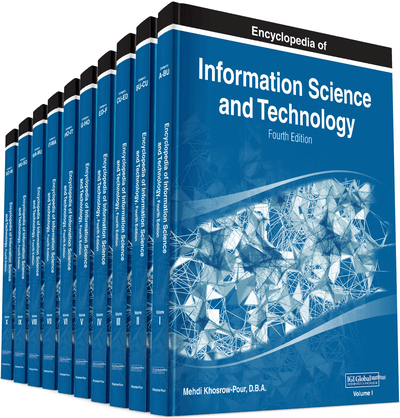# Computer Information Library Clusters

Fu Yuhua (CNOOC Research Institute, China)
DOI: 10.4018/978-1-5225-2255-3.ch382

## Abstract

Based on creating generalized and hybrid set and library with neutrosophy and quad-stage method, this paper presents the concept of “computer information library clusters” (CILC). There are various ways and means to form CILC. For example, CILC can be considered as the “total-library”, and consists of several “sub-libraries”. As another example, in CILC, a “total-library” can be set up, and a number of “sub-libraries” are side by side with the “total-library”. Specially, for CILC, the operation functions can be added; for example, according to “natural science computer information library clusters” (natural science CILC), and applying “variation principle of library (or sub-library)”, “partial and temporary unified theory of natural science so far” with different degrees can be established. Referring to the concept of “natural science CILC”, the concepts of “social science CILC”, “natural science and social science CILC”, and the like, can be presented. While, referreing to the concept of “computer information library clusters”, the concepts of “computer and non-computer information library clusters”, “earth information library clusters”, “solar system information library clusters”, “Milky Way galaxy information library clusters”, “universe information library clusters”, and the like, can be presented.
Chapter Preview
Top

## Constitution Of “Computer Information Library Clusters” (Cilc)

There are various ways and means to form CILC. For instance, CILC can be considered as the “total-library”, and consists of several “sub-libraries”. The example is the “Four-library” presented in (Fu, 2016a) (including four sub-libraries: information library, question library, correlation library, and achievement library). As another example, in CILC, a “total-library” can be set up, and a number of “sub-libraries” are side by side with the “total-library”. In which, the relationships between “total-library” and “sub-libraries” are also various. In (Fu, 2016a), the “total-library” may include all or part of the contents (such as directory, abstracts, etc) of sub-libraries. Of course, generally the “total-library” includes part of the contents of sub-libraries only.

## Key Terms in this Chapter

Partial and Temporary Unified Variation Principle of Natural Science So Far and Partial and Temporary Unified Theory of Natural Science So Far: For unified dealing with the problems of natural science, applying least square method, “partial and temporary unified theory of natural science so far” can be expressed in the form of “partial and temporary unified variation principle of natural science so far”. In this way, the theory of everything to express all of natural laws, described by Hawking that a single equation could be written on a T-shirt, is partially and temporarily realized in the form of “partial and temporary unified variation principle of natural science so far”.

Computer Information Library Clusters (CILC): The expanded and developed result of libraries related to computer information is defined as “computer information library clusters” (CILC). For example, CILC can be considered as the “total-library”, and consists of several “sub-libraries”. As another example, in CILC, a “total-library” can be set up, and a number of “sub-libraries” are side by side with the “total-library”.

Variation Principle of Library: The meaning of “variation principle of library” is the variation principle formed by using least square method (LSM) to process all equations and formulas included in the library. It can pave the way for many practical applications, such as big data analysis and the like.

## Complete Chapter List

Search this Book:
Reset